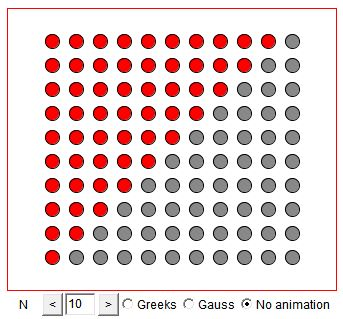## 1 + 2 + 3 + ... + N = N(N+1)/2

### If you are reading this, your browser is not set to run Java applets. Try IE11 or Safari and declare the site https://www.cut-the-knot.org as trusted in the Java setup.What if applet does not run?

The applet attempts to represent in a dynamic form probably the two most famous proofs without words of the formula for triangular numbers. One was yet known to the ancient Greeks, the other was an invention of precocious Gauss. As a story goes, when Gauss was about ten years old, a teacher in the arithmetic class asked his pupils to write down and then add up the numbers from 1 through 100. No sooner the teacher finished stating the exercise when young Gauss placed his slate on the teacher's desk. Later on he explained how he managed to get the result so fast. He came up with the method we used elsewhere in a discussion on all kinds of numbers.

Gauss' method is applicable to computing the sum of any arithmetic progression (or series):

 a, a + d, a + 2d, ..., a + nd (ak = a + kd).

The easiest way to compute the sum of the consecutive terms is to add two copies of the series - one written in reverse order - term by term and sum up:

 a + (a + d) + (a + 2d) + ... + (a + nd) (a + nd) + (a + (n-1)d) + (a + (n-2)d) + ... + a (2a + nd) + (2a + nd) + (2a + nd) + ... + (2a + nd),

which leads to the sum of (n + 1) terms all equal to (2a + nd). Since

 2a + nd = a + (a + nd) = a0 + an,

we write, for the arithmetic series,

 a + (a + d) + (a + 2d) + ... + (a + nd) = (n + 1)(a0 + an)/2.

For the sum of the whole numbers, a = 0 and d = 1, so that a0 = 0 and an = n. The sum is therefore

 (1) 1 + 2 + ... + n = n(n + 1)/2,

as expected.

The derivation is so common and by now so routine that I do not think anybody nowadays computes the foregoing sums in any other way. Thus I cannot even start describing my surprise on receiving a letter from Monty Phister:

 Dear Alex, Two local young ladies ages 14 and 15 were given the problem 'add all the integers from 1 to 100' and were told to do it by looking for patterns. Some of their classmates found Gauss's solution. Some came with an answer trivial mathematically, but I thought it was interesting. They noticed the sum of integers 1 to 10 is 55, from 11 to 20 is 155, from 21 to 30 is 255. They inferred that each sum was 100 greater than the one before, so the sum from 1 to 100 is

 (2) 55+155+255+355+455+555+655+755+855+955=5050

 If one uses n instead of 10, the difference between one sum and the next is n squared. For example the sum of integers 1 to 7 is 28, from 8 to 14 is 77 (49 greater than 28), from 15 to 21 is 126 (49 greater than 77) and so on. Monty

Note that the sum in (2) can be evaluated with the basic shortcut (1):

 55+155+255+355+455+555+655+755+855+955 =   10·55 + (0 + 100 + 200 + ... + 900) =   550 + 100·(1 + 2 + ... + 9) =   550 + 100·9·10/2 =   550 + 100·45 =   550 + 4500 =   5050.

Thus the value of the observation (2) may be questioned. But, as Monty has noted, (2) conceals a pattern not obvious in (1). If nothing else, noticing this pattern provides for an extra exercise. I would be delighted if my students went that far. It is quite wonderful when students do such significant (even if simple) mathematics on their own.

(Monty later informed me that it all happened at the Pojoaque Valley High School, NM. The teacher was Lanse Carter and the students were Desiree Martinez and Amber Lopez).

### References

1. H. W. Eves, In Mathematical Circles, MAA, 2002Copyright © 1996-2018 Alexander Bogomolny

 65250609